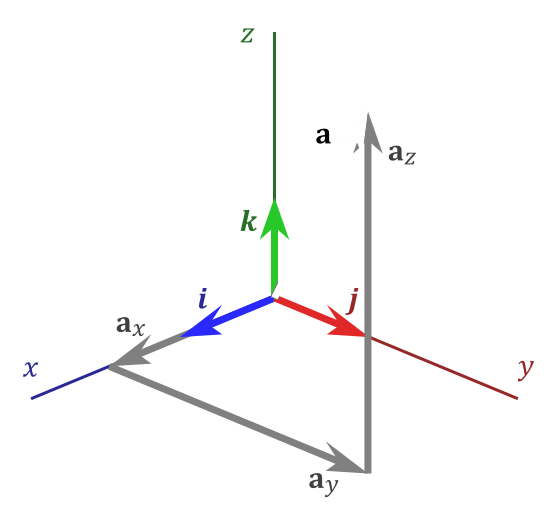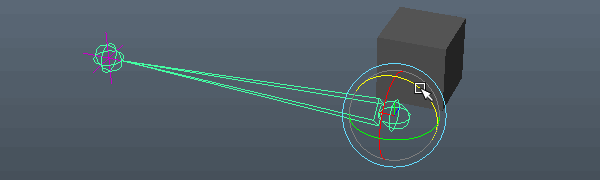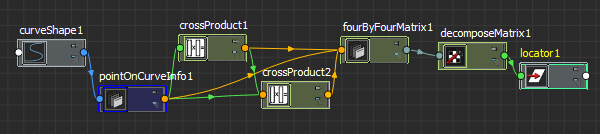# Stumbling Toward 'Awesomeness'

A Technical Art Blog

## Friday, April 6, 2018

### Vector Math Examples in UE4 Python

One of the most visited/indexed posts on this site was the brief Maya vector math post, now that we are releasing Python in UE4, I thought I would port those examples to UE4 Python.

## Creating Vectors

Let’s query the bounding box extents of a skeletal mesh actor. This will return two points in world space as vectors:

```bbox = mesh.get_actor_bounds(False) v1, v2 = bbox, bbox```

If we print the first vector, we see it’s a struct of type Vector

```print v1 #<Struct 'Vector' (0x000001EACECEFE78) {x: 540.073303, y: 32.021194, z: 124.710869}>;```

If you want the vector as a tuple or something to export to elsewhere, you just make a tuple of its components:

```print (v1.x, v1.y, v1.z) #(540.0733032226562, 32.02119445800781, 124.71086883544922)```

If you want to create your own vector, you simply do:

```my_vec3 = unreal.Vector(1,2,3) Print my_vec3 #<Struct 'Vector' (0x000001EACECECA40) {x: 1.000000, y: 2.000000, z: 3.000000}>```

## Length / Distance / Magnitude

The Vector struct supports many mathematical operations, let’s say we want to get the distance from v1 to v2, we would subtract them, which returns a new vector, then we would get the length (‘size’ in this case) of the vector:

```new_vec = v2-v1 Print new_vec.size() #478.868011475```

The vector struct has a lot of convenience functions built in (check out the docs here) , for instance, let’s get the distance from v1, to v2 (the diagonal across our bounding box), without doing the above by calling the dist() function:

```print v1.dist(v2) #478.868011475```

There is also a distSquared function it you just want to quickly compare which are greater and not calc real distance.

## USE CASE: FIND SMALL ACTORS

Using what we got, let’s play in the default scene:We can iterate through the scene and find actors under a certain size/volume:

```for actor in static_meshes: mesh_component = actor.get_component_by_class(unreal.StaticMeshComponent) v1, v2 = mesh_component.get_local_bounds() if v1.dist(v2) < 150: print actor.get_name() #Statue```

I am querying the bounds of the static mesh components because the get_bounds() on the actor class returns the unscaled bounds, you may notice that many things in the scene, including the statue and floor are scaled. This is reflected in the get_local_bounds() of the mesh component.

## DOT PRODUCT / ANGLE BETWEEN TWO VECTORS

If we wanted to know the angle between two vectors, we would use the dot product of those, let’s create two new vectors v1, and v2, because our previous were not really vectors per se, but point locations Just like in UE4, you use the ‘|’ pipe to do a vector dot product.

```v1 = unreal.Vector(0,0,1) v2 = unreal.Vector(0,1,1) v1.normalize() v2.normalize() print v1 | v2 print v1 * v2```

Notice that asterisk will multiply the vectors, pipe will return the sum of the components, or the dot product / scalar product as a float.

For the angle between, let’s import python’s math library:

```import math dot = v1|v2 print math.acos(dot) #returns 0.785398180512 print math.acos(dot) * 180 / math.pi #returns 45.0000009806```

Above I used the Python math library, but there’s also an Unreal math library available unreal.MathLibrary, you should check that out, it has vector math functions that don’t exist in the vector class:

```dot = v1|v2 print unreal.MathLibrary.acos(dot) #returns 0.785398180512 print unreal.MathLibrary.acos(dot) * 180 / math.pi #returns 45.0000009806 print unreal.MathLibrary.radians_to_degrees(unreal.MathLibrary.acos(dot)) #returns: 45.0```

## USE CASE: CHECK ACTOR COLINEARITY

Let’s use the dot product to check if chairs are colinear, or facing the same direction. Let’s dupe one chair and call it ‘Chair_dupe’:```fwd_vectors = {} for actor in static_meshes: if 'Chair' in actor.get_name(): actor_vec = actor.get_actor_forward_vector() for stored_actor in fwd_vectors: if actor_vec | fwd_vectors[stored_actor] == 1.0: print actor.get_name(), 'is colinear to', stored_actor fwd_vectors[actor.get_name()] = actor_vec #returns: Chair_dupe is colinear to Chair```

I hope this was helpful, I can post some other examples later.

posted by Chris at 1:23 AM

## BEFORE WE BEGIN

This post is about how to use vector math and trigonometric functions in Maya, it is not a linear algebra or vector math course, it should give you what you need to follow along in Maya while you learn with online materials. Kahn Academy is a great online learning resource for math, and Mathematics for Computer Graphics, and Linear Algebra and its Applications are very good books. Gilbert Strang, the Author of Linear Algebra, has his entire MIT Linear Algebra course lectures here in video form. Also, Volume 2 of Complete Maya Programming has some vector math examples in MEL and C++.## VECTORS

Think of the white vector above as a movement. It does have three scalar values (ax, ay, az), sure, but do not think of a vector as a point or a position. When you see a vector, I believe it helps to imagine it as a movement from 0,0,0 – an origin. We don’t know where it started, we only know the movement.

A vector has been normalized, or is considered a unit vector, when it’s length is one. This is achieved by dividing each component by the length.

## VECTOR LIBRARIES

There are many Python libraries dedicated to vector math, but none ship with Python itself. I have tried numPy, then pyEuclid, and finally piMath. It can definitely be a benefit to load the same vector class across multiple apps like Maya, MotionBuilder, etc.. But, I used those in a time when MotionBuilder had no vector class, and before Maya had the API. Today, I use the vector class built into the Maya Python API (2.0), which wraps the underlying Maya C++ code: MVector

I had to call out 2.0 above, as those of you using the old API, you have to ‘cast’ your vectors to/from, meaning that classes like MVector (Maya’s vector class) don’t accept python objects like lists or tuples, this is still the case with the 2014 SWIG implementation of the default API, but not API 2.0. One solution is to override the MVector class in a way that it accepts a Python lists and tuples, essentially automatically casting it for you:

```class MVector(om.MVector):     def __init__(self, *args):         if( issubclass, args, list ) and len(args)== 3:             om.MVector.__init__(self, args, args, args)         else:             om.MVector.__init__(self, args)```

But that aside, just use Maya Python API 2.0:

```#import API 2.0 import maya.api.OpenMaya as om #import old API import maya.OpenMaya as om_old```

## CREATING VECTORS IN MAYA

Let’s first create two cubes, and move them

```import maya.cmds as cmds import maya.api.OpenMaya as om cube1, cube2 = cmds.polyCube(), cmds.polyCube() cmds.xform(cube2, t=(1,2,3)) cmds.xform(cube1, t=(3,5,2))```

Let’s get the translation of each, and store those as MVectors

```t1, t2 = cmds.xform(cube1, t=1, q=1), cmds.xform(cube2, t=1, q=1) print t1,t2 v1, v2 = om.MVector(t1), om.MVector(t2) print v1, v2```

This will return the translation in the form [x, y, z], and also the MVector, which will print: (x, y, z), and in the old API: <__main__.MVector; proxy of <Swig Object of type ‘MVector *’ at 0x000000002941D2D0> >. This is a SWIG wrapped C++ object, API 2.0 prints the vector.

Note: I just told you to think of vectors as a movement, and not as a position, and the first example I give stores translation in a vector. Maybe not the best, but remember this translation, is really being stored as a movement in space from an origin.

So let’s start doing stuff and things.

## LENGTH / DISTANCE / MAGNITUDE

We have two translations, both stored as vectors, let’s get the distance between them, to do this, we want to make a new vector that describes a ray from one location to the other and then find it’s length, or magnitude. To do this we subtract each component of v1 from v2:

```v = v2-v1 print v```

This results in ‘-2.0 -3.0 1.0’.

To get the length of the vector we actually get the square root of the sum of x,y,and z squared sqrt(x^2+y^2+z^2), but as we haven’t covered the math module yet, let’s just ask the MVector for the ‘length’:

`print om.MVector(v2-v1).length()`

This will return 3.74165738677, which, if you snap a measure tool on the cubes, you can verify:## Use Case: Distance Check

As every joint in a hierarchy is in it’s parent space, a joint’s ‘magnitude’ is it’s length. Let’s create a lot of joints, then select them by joint length.

```import maya.cmds as cmds import random as r import maya.api.OpenMaya as om   root = cmds.joint() jnts = []   for i in range(0, 2000): cmds.select(cl=1) jnt = cmds.joint() trans = (r.randrange(-100,100), r.randrange(-100,100), r.randrange(-100,100)) cmds.xform(jnt, t=trans) jnts.append(jnt)   cmds.parent(jnts, root)```So we’ve created this cloud of joints, but let’s just select those joints with a joint length of less than 50.

```sel = [] for jnt in jnts: v = om.MVector(cmds.xform(jnt, t=1, q=1)) if v.length() < 50: sel.append(jnt)   cmds.select(sel)```

## DOT PRODUCT / ANGLE BETWEEN TWO VECTORS

The dot product is a scalar value obtained by performing a specific operation on two vector components. This doesn’t make much sense, so I will tell you that the dot product is extremely useful in finding the angle between two vectors, or checking which general direction something is pointing.

```dot = v1*v2 print dot```

## USE CASE: Direction TestThe dot product of two normalized vectors will always be between -1.0 and 1.0, if the dot product is greater than zero, the vectors are pointing in the same general direction, zero means they are perpendicular, less than zero means opposite directions. So let’s loop through our joints and select those that are facing the x direction:

```sel = [] for jnt in jnts: v = om.MVector(cmds.xform(jnt, t=1, q=1)).normal() dot = v*om.MVector([1,0,0]) if dot > 0.7: sel.append(jnt) cmds.select(sel)```

## USE CASE: Test World Colinearity

This one comes from last week in the office, one of my guys wanted to know how to check which way in the world something was facing. I believe it was to derive some information from arbitrary skeletons. This builds on the above by getting each vector of a node in world space.

```def getLocalVecToWorldSpace(node, vec=om.MVector.kXaxisVector): matrix = om.MGlobal.getSelectionListByName(node).getDagPath(0).inclusiveMatrix() vec = (vec * matrix).normal() return vec     def axisVectorColinearity(node, vec): vec = om.MVector(vec)   x = getLocalVecToWorldSpace(node, vec=om.MVector.kXaxisVector) y = getLocalVecToWorldSpace(node, vec=om.MVector.kYaxisVector) z = getLocalVecToWorldSpace(node, vec=om.MVector.kZaxisVector)   #return the dot products return {'x': vec*x, 'y':vec*y, 'z':vec*z}   jnt = cmds.joint() print axisVectorColinearity(jnt, [0,0,1])```

You can rotate the joint around and you will see which axis is most closely pointing to the world space vector you have given as an input.

## USE CASE: Angle Between VectorsWhen working with unit vectors, we can get the arc cosine of a dot product to derive the angle between the two vectors, but this requires trigonometric functions, which are not available in our vector class, for this we must import the math module. Scratching the code above, let’s find the angle between two joints:

```import maya.cmds as cmds import maya.api.OpenMaya as om import math   jnt1 = cmds.joint() cmds.select(cl=1) jnt2 = cmds.joint() cmds.xform(jnt2, t=(0,0,10)) cmds.xform(jnt1, t=(10,0,0)) cmds.select(cl=1) root = cmds.joint() cmds.parent([jnt1, jnt2], root)   v1 = om.MVector(cmds.xform(jnt1, t=1, q=1)).normal() v2 = om.MVector(cmds.xform(jnt2, t=1, q=1)).normal()   dot = v1*v2 print dot print math.acos(dot) print math.acos(dot) * 180 / math.pi```

So at the end here, the arc Cosine of the dot product returns the angle in radians (1.57079632679), which we convert to degrees by multiplying it by 180 and dividing by pi (90.0). To check your work, there is no angle tool in Maya, but you can create a circle shape and set the sweep degrees to your result.

Now that you know how to convert radians to an angle, if you store the result of the above in an MAngle class, you can ask for it however you like:

`print om.MAngle(math.acos(dot)).asDegrees()`

Now that you know how to do this, there is an even easier, using the angle function of the MVector class, you can ask it the angle given a second vector:

`print v1.angle(v2)`

There are also useful attributes v1.rotateBy(r,r,r) for an offset and v1.rotateTo(v2). I say (r,r,r) in my example, but the rotateBy attr takes angles or radians.

## USE CASE: Orient-DriverMoving along, let’s apply these concepts to something more interesting. Let’s drive a blendshape based on orientation of a joint. Since the dot product is a scalar value, we can pipe this right into a blendshape, when the dot product is 1.0, we know that the orientations match, when it’s 0, we know they are perpendicular.We will use a locator constrained to the child to help in deriving a vector. The fourByFourMatrix stores the original position of the locator. I tried using the holdMatrix node, which should store a cached version of the original locator matrix, but it kept updating. (?) We use the vectorProduct node in ‘dot product’ mode to get the dot product of the original vector and the current vector of the joint. We then pipe this value into the weight of the blendshape node.

Now, this simple example doesn’t take twist into account, and we aren’t setting a falloff or cone, the falloff will be 1.0 when the vectors align and the blendshape is on 100% and 0.0, when they’re perpendicular and the blendshape will be on 0%. I also don’t clamp the dot product, so the blendshape input can go to -1.

## CROSS PRODUCT / PERPENDICULAR VECTOR TO TWO VECTORS

The cross product results in a vector that is perpendicular to two vectors. Generally you would do (v1.y*v2.z-v1.z*v2.y, v1.z*v2.x-v1.x*v2.z, v1.x*v2.y-v1.y*v2.x), ut luckily, the vector class manages this for us by using the ‘^’ symbol:

```cross = v1^v2 print cross```

## USE CASE: Building a coordinate frameIf we get the cross product of v1^v2 above, and use this vector to now cross (v1 x v2)x v1, we will now have a third perpendicular vector to build a coordinate system or ‘orthonormal basis’. A useful example would be aligning a node to a nurbs curve using the pointOnCurveInfo node.In the example above, we are using two cross products to build a matrix from the tangent of the pointOnCurveInfo and it’s position, then decomposing this matrix to set the orientation and position of a locator.

Many people put content like this behind a paywall.